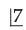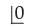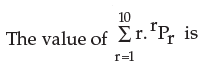Courses

# Test: Basic Concepts Of Permutations And Combinations- 1

## 40 Questions MCQ Test Business Mathematics and Logical Reasoning & Statistics | Test: Basic Concepts Of Permutations And Combinations- 1

Description
This mock test of Test: Basic Concepts Of Permutations And Combinations- 1 for CA Foundation helps you for every CA Foundation entrance exam. This contains 40 Multiple Choice Questions for CA Foundation Test: Basic Concepts Of Permutations And Combinations- 1 (mcq) to study with solutions a complete question bank. The solved questions answers in this Test: Basic Concepts Of Permutations And Combinations- 1 quiz give you a good mix of easy questions and tough questions. CA Foundation students definitely take this Test: Basic Concepts Of Permutations And Combinations- 1 exercise for a better result in the exam. You can find other Test: Basic Concepts Of Permutations And Combinations- 1 extra questions, long questions & short questions for CA Foundation on EduRev as well by searching above.
QUESTION: 1

Solution:
QUESTION: 2

Solution:
QUESTION: 3

###is equal to

Solution:
QUESTION: 4is a symbol equal to

Solution:
QUESTION: 5

In nPr, n is always

Solution:
QUESTION: 6

In nPr , the restriction is

Solution:
QUESTION: 7

In nPr = n (n–1) (n–2) ………………(n–r–1), the number of factor is

Solution:
QUESTION: 8

nPr can also written as

Solution:
QUESTION: 9

If nP4 = 12 × nP2, the n is equal to

Solution:
QUESTION: 10

If . nP3 : nP2 = 3 : 1, then n is equal to

Solution:
QUESTION: 11

m+nP2 = 56, m–nP2 = 30 then

Solution:
QUESTION: 12

If  5Pr = 60, then the value of r is

Solution:
QUESTION: 13

If n1+n2P2 = 132, n1–n2P2 = 30 then,

Solution:
QUESTION: 14

The number of ways the letters of the word COMPUTER can be arranged is

Solution:

Since all letters in the word "COMPUTER" are distinct then the arrangements is 8! = 8 x 7 x 6 x 5 x 4 x 3 x 2 x 1 = 40320

QUESTION: 15

The number of arrangements of the letters in the word FAILURE, so that vowels are always coming together is

Solution:
QUESTION: 16

10 examination papers are arranged in such a way that the best and worst papers never come together. The number of arrangements is

Solution:

No. of ways in which 10 paper can arrange is 10! Ways.
When the best and the worst papers come together, regarding the two as one paper, we have only 9 papers.
These 9 papers can be arranged in 9! Ways.
And two papers can be arranged themselves in 2! Ways.
No. of arrangement when best and worst paper do not come together,
= 10!- 9!.2! = 9!(10-2) = 8.9!.

QUESTION: 17

n articles are arranged in such a way that 2 particular articles never come together. The number of such arrangements is

Solution:
QUESTION: 18

If 12 school teams are participating in a quiz contest, then the number of ways the first, second and third positions may be won is

Solution:
QUESTION: 19

The sum of all 4 digit number containing the digits 2, 4, 6, 8, without repetitions is

Solution:
QUESTION: 20

The number of 4 digit numbers greater than 5000 can be formed out of the  digits 3,4,5,6 and 7(no. digit is repeated). The number of such is

Solution:
QUESTION: 21

4 digit numbers to be formed out of the figures 0, 1, 2, 3, 4 (no digit is repeated) then number of such numbers is

Solution:
QUESTION: 22

The number of ways the letters of the word “Triangle” to be arranged so that the word ’angle’ will be always present is

Solution:
QUESTION: 23

If the letters word ‘Daughter ’ are to be arranged so that vowels occupy the odd places, then number of different words are

Solution:

There are only 3 vowels, a, u, and e, and there are 4 odd places.  So
one of the odd spaces must contain a consonant.
We can choose 3 of the 4 odd places to put the vowels in C(4,3) or 4 ways.
For each of those vowel , we can  rearrange them  in P(3,3) or 3! or 6 ways.
And we can rearrange the 5 consonants in P(5,5) or 5! or 120 ways.
Hence the total number of different words formed are 6*4*120 = 2880

QUESTION: 24

The number of ways in which 7 girls form a ring is

Solution:
QUESTION: 25

The number of ways in which 7 boys sit in a round table so that two particular boys may sit together is

Solution:
QUESTION: 26

In how many ways can 10 examination papers be arranged so that the best and the worst papers never come together?

Solution:

No. of ways in which 10 paper can arranged is 10! Ways.
When the best and the worst papers come together, regarding the two as one paper, we have only 9 papers.
These 9 papers can be arranged in 9! Ways.
And two papers can be arranged themselves in 2! Ways.
No. of arrangement when best and worst paper do not come together,
= 10! - 9! × 2!
= 9!(10 - 2)
= 8 × 9!

QUESTION: 27

3 ladies and 3 gents can be seated at a round table so that any two and only two of the ladies sit together. The number of ways is

Solution:
QUESTION: 28

The number of ways in which the letters of the word DOGMATIC can be arranged is

Solution:
QUESTION: 29

The number of arrangements of 10 different things taken 4 at a time in which one particular thing always occurs is

Solution:
QUESTION: 30

The number of permutations of 10 different things taken 4 at a time in which one particular thing never occurs is

Solution:
QUESTION: 31

Mr. X and Mr. Y enter into a railway compartment having six vacant seats. The number of ways in which they can occupy the seats is

Solution:
QUESTION: 32

The number of numbers lying between 100 and 1000 can be formed with the digits 1, 2, 3, 4, 5, 6, 7 is

Solution:
QUESTION: 33

The number of numbers lying between 10 and 1000 can be formed with the digits 2,3,4,0,8,9 is

Solution:
QUESTION: 34

In a group of boys the number of arrangement of 4 boys is 12 times the number of arrangements of 2 boys. The number of boys in the group is

Solution:
QUESTION: 35Solution:
QUESTION: 36

The total number of 9 digit numbers of different digits is

Solution:
QUESTION: 37

The number of ways in which 6 men can be arranged in a row so that the particular 3 men sit together, is

Solution:
QUESTION: 38

There are 5 speakers A, B, C, D and E. The number of ways in which A will speak always before B is

Solution:
QUESTION: 39

There are 10 trains plying between Calcutta and Delhi. The number of ways in which a person can go from Calcutta to Delhi and return by a different train is

Solution:
QUESTION: 40

The number of ways in which 8 sweets of different sizes can be distributed among 8 persons of different ages so that the largest sweat always goes to be younger assuming that each one of then gets a sweet is

Solution:

The number of ways in which 8 sweets of different sizes can be distributed among 8 persons of different ages so that the largest sweat goes to the younger assuming that each one of them gets a sweet.
Since the largest goes to the youngest, that leaves 7 sweets  to distribute to 7 people.

7P7 = 7! = 5040 ways